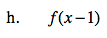### Home > CAAC > Chapter 12 > Lesson 12.4.2 > Problem12-76

12-76.
1. For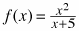and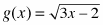find the following, if possible. Homework Help ✎

1. f(6)

2. g(17)

3. f(−5)

4. g(−2)

5. g(−1) − f(2)

6. f(4) + g(2)

7. g(x + 2)

8. f(x − 1)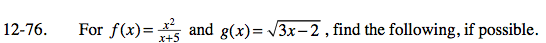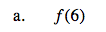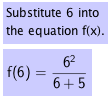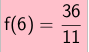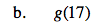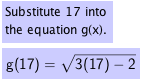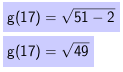g(17) = 7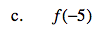f( -5 ) = undefined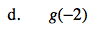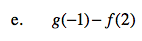Substitue -1 into the equation g(x) and 2 into the equation f(x).

Subtract the second answer from the first.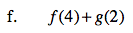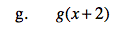$g( x + 2 ) = \sqrt{3x + 4}$